Electron. J. Differential Equations, Vol. 2020 (2020), No. 117, pp. 1-16.

### Existence and nonexistence of radial solutions for semilinear equations with bounded nonlinearities on exterior domains Joseph Iaia

Abstract:
In this article we study radial solutions of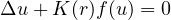on the exterior of the ball of radius R>0 centered at the origin in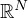, where f is odd with f<0 on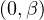, f>0 on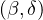,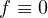for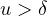, and where the function K(r) is assumed to be positive and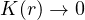as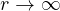. The primitive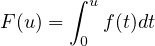has a hilltop'' at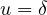. With mild assumptions on f we prove that if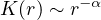with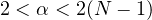then there are n solutions ofon the exterior of the ball of radius R such that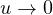asif R>0 is sufficiently small. We also show there are no solutions if R>0 is sufficiently large.

Submitted January 6, 2020. Published December 1, 2020.
Math Subject Classifications: 34B40, 35B05.
Key Words: Sublinear equation; radial solution; exterior domain.

Show me the PDF file (371 KB), TEX file for this article.Joseph A. Iaia Department of Mathematics University of North Texas, P.O. Box 311430 Denton, TX 76203-5017, USA email: iaia@unt.edu

Return to the EJDE web page